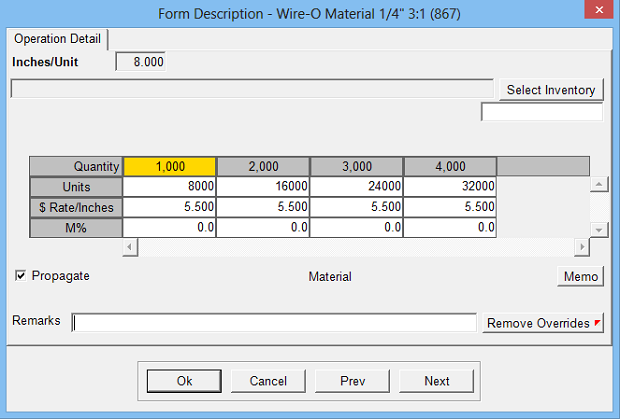Estimates the cost of material by the linear inch.

Operation Setup Requirements:
1. Description
2. Rate: Cost per linear inch (or thousand inches)
3. Sell \$ use: Markup % (recommended) or alternate sell rate
4. Calc: 33
5. Sub Key: Material
6. Rate Per M: Makes rate cost per thousand linear inches
8. Set Inches per Unit to: Option to default to Zero, Finish Length or Finish Width
9. Round Inches up to nearest optionEstimate Specification Input Prompts:

1. Inches/Unit (not editable if set to form dimension)
2. Units
3. Rate
4. M%
5. Remarks
6. MemoCalculation:

Units = Inches per Unit x Quantity

Units x Rate = Cost \$

Cost \$ x Markup % = Markup \$

Cost \$ + Markup \$ = Sell \$